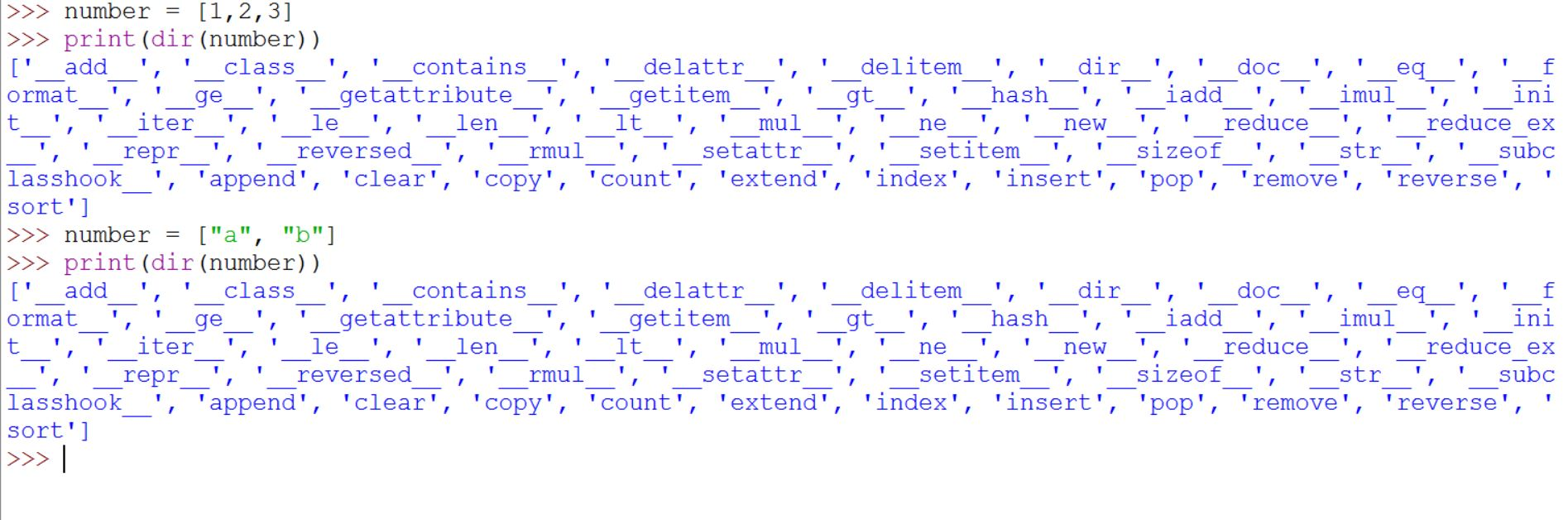Open in App
Not now

# reflection in Python

• Difficulty Level : Hard
• Last Updated : 17 Nov, 2020

Reflection refers to the ability for code to be able to examine attributes about objects that might be passed as parameters to a function. For example, if we write type(obj) then Python will return an object which represents the type of obj.

Using reflection, we can write one recursive reverse function that will work for strings, lists, and any other sequence that supports slicing and concatenation. If an obj is a reference to a string, then Python will return the str type object. Further, if we write str() we get a string which is the empty string. In other words, writing str() is the same thing as writing “”. Likewise, writing list() is the same thing as writing [].

 `# Python program to illustrate reflection ``def` `reverse(sequence): ``    ``sequence_type ``=` `type``(sequence) ``    ``empty_sequence ``=` `sequence_type() ``     ` `    ``if` `sequence ``=``=` `empty_sequence: ``        ``return` `empty_sequence ``     ` `    ``rest ``=` `reverse(sequence[``1``:]) ``    ``first_sequence ``=` `sequence[``0``:``1``] ``     ` `    ``# Combine the result ``    ``final_result ``=` `rest ``+` `first_sequence``     ` `    ``return` `final_result `` ` `# Driver code ``print``(reverse([``10``, ``20``, ``30``, ``40``])) ``print``(reverse(``"GeeksForGeeks"``)) `

Output:

```[40, 30, 20, 10]
skeeGroFskeeG
```

Reflection-enabling functions

Reflection-enabling functions include type(), isinstance(), callable(), dir() and getattr().

1. type and isinstance – Refer here
2. Callable() :A callable means anything that can be called. For an object, determines whether it can be called. A class can be made callable by providing a __call__() method. The callable() method returns True if the object passed appears callable. If not, it returns False.
Examples:

```x = 5

def testFunction():
print("Test")

y = testFunction

if (callable(x)):
print("x is callable")
else:
print("x is not callable")

if (callable(y)):
print("y is callable")
else:
print("y is not callable")
```

Output:

```x is not callable
y is callable```

callable when used in OOP

```class Foo1:
def __call__(self):
print('Print Something')

print(callable(Foo1))
```

Output:

`True`
3. Dir : The dir() method tries to return a list of valid attributes of the object. The dir() tries to return a list of valid attributes of the object.
If the object has __dir__() method, the method will be called and must return the list of attributes.
If the object doesn’t have __dir()__ method, this method tries to find information from the __dict__ attribute (if defined), and from type object. In this case, the list returned from dir() may not be complete.

Examples:

```number = [1,2,3]
print(dir(number))

characters = ["a", "b"]
print(dir(number))```

Output:4. Getattr : The getattr() method returns the value of the named attribute of an object. If not found, it returns the default value provided to the function.The getattr method takes three parameters object, name and default(optional).
```class Employee:
salary = 25000
company_name= "geeksforgeeks"

employee = Employee()
print('The salary is:', getattr(employee, "salary"))
print('The salary is:', employee.salary)```

Output:

```The salary is: 25000
The salary is: 25000```